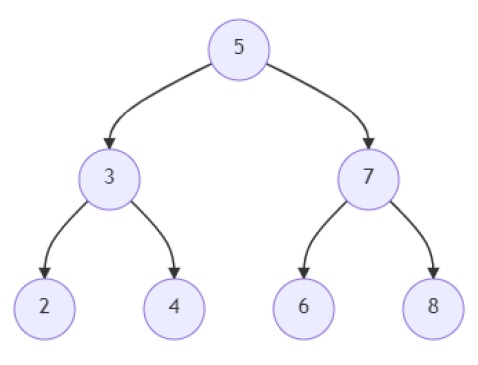# Program to find out distance between two nodes in a binary tree in Python

Suppose, we are given a binary tree and are asked to find the distance between two nodes in the binary tree. We find out the edges between the two nodes like in a graph and return the number of edges or the distance between them. A node of a tree has the structure as below −

data : <integer value>
right : <pointer to another node of the tree>
left : <pointer to another node of the tree>

So, if the input is likeand the nodes between which we have to find the distance between are 2 and 8; then the output will be 4.

The edges between the two nodes 2 and 8 are: (2, 3), (3, 5), (5, 7), and (7, 8). There are 4 edges in the path between them, so the distance is 4.

To solve this, we will follow these steps −

• Define a function findLca() . This will take root, p, q
• if root is same as null, then
• return null
• if data of root is any of (p,q), then
• return root
• left := findLca(left of root, p, q)
• right := findLca(right of root, p, q)
• if left and right is not null, then
• return root
• return left or right
• Define a function findDist() . This will take root, data
• queue := a new deque
• insert a new pair (root, 0) at the end of queue
• while queue is not empty, do
• current := first value of the leftmost pair in queue
• dist := second value of the leftmost pair in queue
• if data of current is same as data, then
• return dist
• if left of current is not null, then
• add pair (left of current, dist+1) to queue
• if right of current is not null, then
• add pair (current.right, dist+1) to queue
• node := findLca(root, p, q)
• return findDist(node, p) + findDist(node, q)

## Example

Let us see the following implementation to get better understanding −

import collections
class TreeNode:
def __init__(self, data, left = None, right = None):
self.data = data
self.left = left
self.right = right

def insert(temp,data):
que = []
que.append(temp)
while (len(que)):
temp = que
que.pop(0)
if (not temp.left):
if data is not None:
temp.left = TreeNode(data)
else:
temp.left = TreeNode(0)
break
else:
que.append(temp.left)

if (not temp.right):
if data is not None:
temp.right = TreeNode(data)
else:
temp.right = TreeNode(0)
break
else:
que.append(temp.right)

def make_tree(elements):
Tree = TreeNode(elements)
for element in elements[1:]:
insert(Tree, element)
return Tree

def search_node(root, element):
if (root == None):
return None

if (root.data == element):
return root

res1 = search_node(root.left, element)
if res1:
return res1

res2 = search_node(root.right, element)
return res2

def print_tree(root):
if root is not None:
print_tree(root.left)
print(root.data, end == ', ')
print_tree(root.right)

def findLca(root, p, q):
if root is None:
return None
if root.data in (p,q):
return root
left = findLca(root.left, p, q)
right = findLca(root.right, p, q)
if left and right:
return root
return left or right

def findDist(root, data):
queue = collections.deque()
queue.append((root, 0))
while queue:
current, dist = queue.popleft()
if current.data == data:
return dist
if current.left: queue.append((current.left, dist+1))
if current.right: queue.append((current.right, dist+1))

def solve(root, p, q):
node = findLca(root, p, q)
return findDist(node, p) + findDist(node, q)

root = make_tree([5, 3, 7, 2, 4, 6, 8])
print(solve(root, 2, 8))

## Input

root = make_tree([5, 3, 7, 2, 4, 6, 8])
print(solve(root, 2, 8))

## Output

4